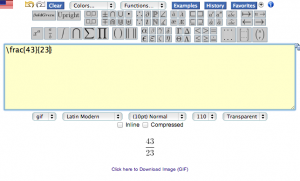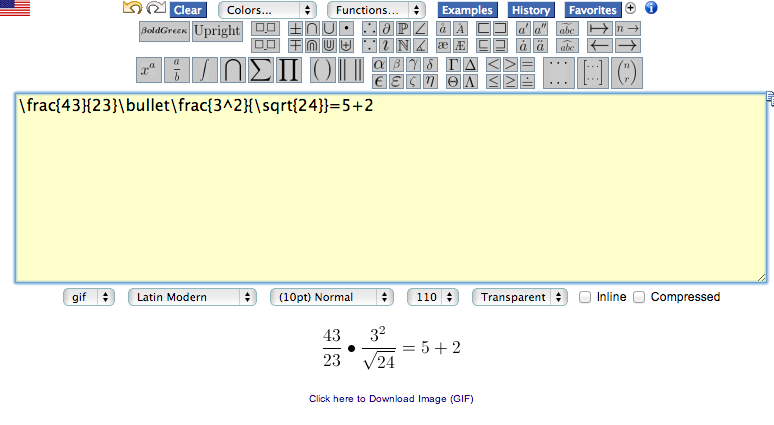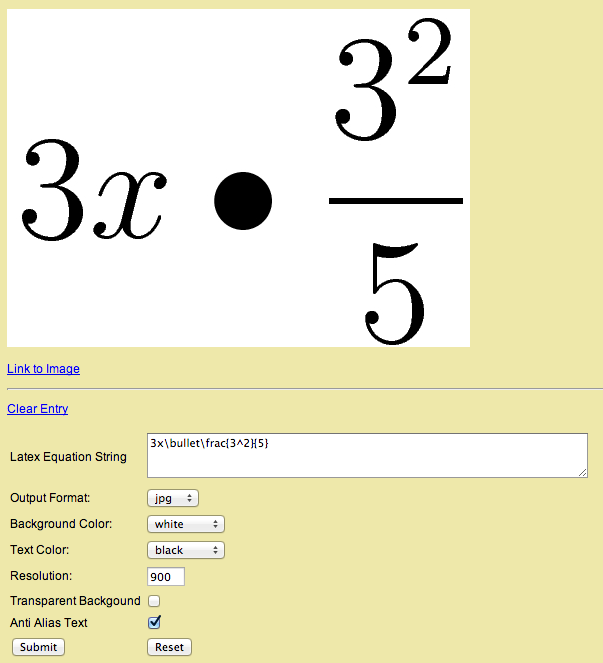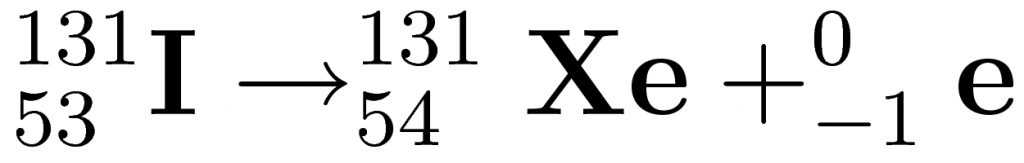# Tutorial for Everyone: LaTeX

There may be times in your life where you need to make a mathematical figure or equation on your computer.

For me, as a high school student, this occurs often in both math and biology. There is no easy way to create a fraction that looks nice in word. Most people use awkward line spacing and tabulation to make fractions, but isn’t there an easier way?

Yup. It’s called LaTeX. LaTeX is basically a markup language that is often used to draw equations.

Start out by going to The Codecogs Equation Editor.

Up above you’ll notice a giant bank of mathematical symbols and templates.

Click one of these and you’ll get some markup in the text box with some empty brackets. Stick some numbers in the brackets and see what happens below the box.Look at that, you’ve created a mathematical figure!

To extend your equation, just keep adding symbols and they’ll fill from left to right. You can also nest these items within each other, so you can have a fraction within a fraction, a number with an exponent within another symbol, and so on.Using this, I bet most of you will be able to figure out how to write some great and useful equations just by playing around with it.Another use I’ve found for LaTeX is in my high school chemistry class. As we discuss radioactive decay, it is necessary to notate isotopes and decay equations on a computer. Luckily, LaTeX is here to save the day.

Lets say that you have an Iodine-131 atom on the left which decays into a Xenon-131 atom and a beta particle. This can be notated in LaTeX with

_{53}^{131}\textrm{\textbf{I}}\rightarrow_{54}^{131}\textrm{\textbf{Xe}}+_{-1}^{0}\textrm{\textbf{e}}

You can probably figure out what all of those things relate to in the result:So there’s your primer on the basics of LaTeX. Enjoy!